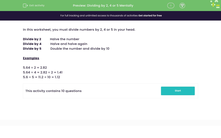# Divide by 2, 4 or 5 Mentally

In this worksheet, students will carry out mental division calculations.Key stage:  KS 3

Curriculum topic:   Number

Curriculum subtopic:   Use Four Operations for All Numbers

Difficulty level:#### Worksheet Overview

In this activity, you will practise dividing numbers by 2, 4 or 5 in your head.

Divide by 2          Halve the number

Divide by 4          Halve and halve again

Divide by 5          Double the number and divide by 10

Examples

5.64 ÷ 2 = 2.82

5.64 ÷ 4 = 2.82 ÷ 2 = 1.41

5.6 ÷ 5 = 11.2 ÷ 10 = 1.12

Does that all make sense?Let's have a go at some questions now.

### What is EdPlace?

We're your National Curriculum aligned online education content provider helping each child succeed in English, maths and science from year 1 to GCSE. With an EdPlace account you’ll be able to track and measure progress, helping each child achieve their best. We build confidence and attainment by personalising each child’s learning at a level that suits them.

Get started